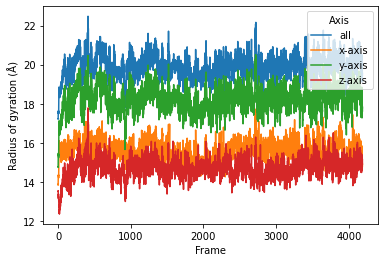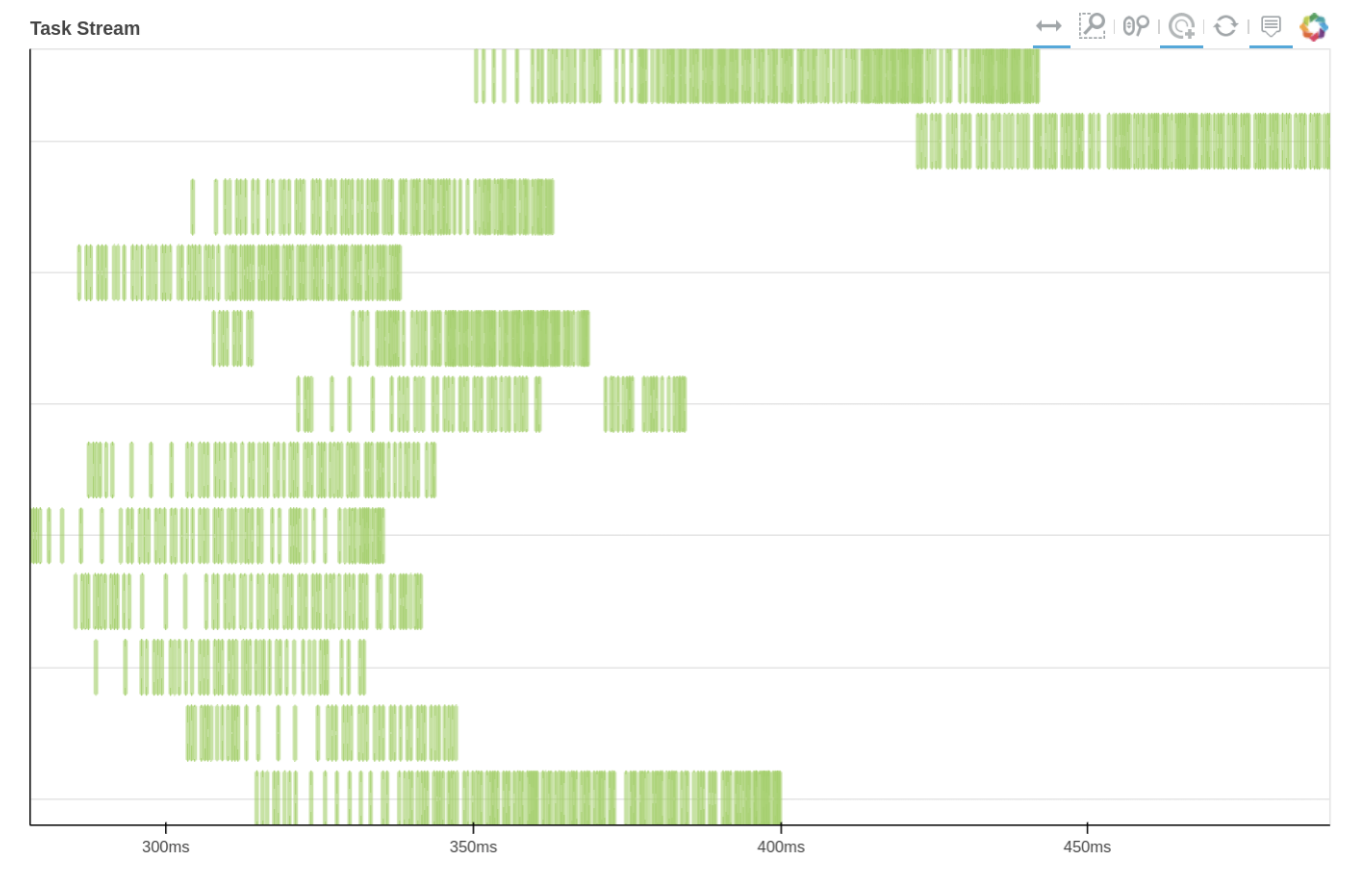# Parallelizing analysis¶

As we approach the exascale barrier, researchers are handling increasingly large volumes of molecular dynamics (MD) data. Whilst MDAnalysis is a flexible and relatively fast framework for complex analysis tasks in MD simulations, implementing a parallel computing framework would play a pivotal role in accelerating the time to solution for such large datasets.

This document illustrates how you can run your own analysis scripts in parallel with MDAnalysis.

Last executed: Sep 23, 2020 with MDAnalysis 2.0.0-dev0

Last updated: August 2020

Minimum version of MDAnalysis: 2.0.0

Packages required:

:

import MDAnalysis as mda

import numpy as np
import pandas as pd
import seaborn as sns
import matplotlib.pyplot as plt
%matplotlib inline


## Background¶

In MDAnalysis, most implemented analysis methods are based on AnalysisBase, which provides a generic API for users to write their own trajectory analysis. However, this framework only takes single-core power of the PC by iterating through the trajectory and running a frame-wise analysis. Below we aim to first explore some possible simple implementations of parallelism, including using multiprocessing and dask. We will also discuss the acceleration approaches that should be considered, ranging from your own multiple-core laptops/desktops to distributed clusters.

The test files we will be working with here feature adenylate kinase (AdK), a phosopho-transferase enzyme. ([BDPW09]). The trajectory has 4187 frames, which will take quite some time to run the analysis on with the conventional serial (single-core) approach.

:

adk = fetch_adk_equilibrium()
protein = u.select_atoms('protein')
print(len(u.trajectory))

4187


For a detail description of this analysis, read Writing your own trajectory.

Here is a common form of single-frame method that we can normally see inside AnalysisBase. It may contain both some dynamic parts that changes along time either implicitly or explicitly (e.g. AtomGroup) and some static parts (e.g. a reference frame).

:

def radgyr(atomgroup, masses, total_mass=None):
# coordinates change for each frame
coordinates = atomgroup.positions
center_of_mass = atomgroup.center_of_mass()

# get squared distance from center
ri_sq = (coordinates-center_of_mass)**2
# sum the unweighted positions
sq = np.sum(ri_sq, axis=1)
sq_x = np.sum(ri_sq[:,[1,2]], axis=1) # sum over y and z
sq_y = np.sum(ri_sq[:,[0,2]], axis=1) # sum over x and z
sq_z = np.sum(ri_sq[:,[0,1]], axis=1) # sum over x and y

# make into array
sq_rs = np.array([sq, sq_x, sq_y, sq_z])

# weight positions
rog_sq = np.sum(masses*sq_rs, axis=1)/total_mass
# square root and return
return np.sqrt(rog_sq)


### Serial Analysis¶

Below is the serial version of the analysis that we normally use.

:

result = []
for frame in u.trajectory:
masses=protein.masses,
total_mass=np.sum(protein.masses)))

:

result = np.asarray(result)

labels = ['all', 'x-axis', 'y-axis', 'z-axis']
serial = pd.DataFrame(result, columns=labels)
serial["Frame"] = serial.index
serial = serial.melt(id_vars="Frame", var_name="Axis",
sns.lineplot(data=serial, x="Frame", y="Radius of gyration (Å)",
hue="Axis")

:

<matplotlib.axes._subplots.AxesSubplot at 0x7fee546fc6d0>## Parallelization in a simple per-frame fashion¶

### Frame-wise form of the function¶

The coordinates of the atomgroup analysed change with each frame of the trajectory. We need to explicitly point the analysis function to the frame that needs to be analysed with a frame_index: atomgroup.universe.trajectory[frame_index] in order to update the positions (and any other dynamic per-frame information) appropriately. Therefore, the first step to making the radgyr function parallelisable is to add a frame_index argument.

:

def radgyr_per_frame(frame_index, atomgroup, masses, total_mass=None):

# index the trajectory to set it to the frame_index frame
atomgroup.universe.trajectory[frame_index]

# coordinates change for each frame
coordinates = atomgroup.positions
center_of_mass = atomgroup.center_of_mass()

# get squared distance from center
ri_sq = (coordinates-center_of_mass)**2
# sum the unweighted positions
sq = np.sum(ri_sq, axis=1)
sq_x = np.sum(ri_sq[:,[1,2]], axis=1) # sum over y and z
sq_y = np.sum(ri_sq[:,[0,2]], axis=1) # sum over x and z
sq_z = np.sum(ri_sq[:,[0,1]], axis=1) # sum over x and y

# make into array
sq_rs = np.array([sq, sq_x, sq_y, sq_z])

# weight positions
rog_sq = np.sum(masses*sq_rs, axis=1)/total_mass
# square root and return
return np.sqrt(rog_sq)


Dask is a flexible library for parallel computing in Python. It provides advanced parallelism for analytics and has been integrated or utilized in many scientific softwares. It can be scaled from one single computer to a cluster of computers inside a HPC center.

Dask has a dynamic task scheduling system with synchronous (single-threaded), threaded, multiprocessing and distributed schedulers. The wrapping function in dask, dask.delayed, mimics for loops and wraps Python code into a Dask graph. This code can then be easily run in parallel, and visualized with dask.visualize() to examine if the task is well distributed. The code inside dask.delayed is not run immediately on execution, but pushed into a job queue waiting for submission. You can read more on dask website.

Comaring to multiprocssing, the downside of multiprocessing is that it is mostly focused on single-machine multicore parallelism (without extra manager). It is hard to operate on multimachine conditions. Below are two simple examples to use Dask to achieve the same task as multiprocessing does.

The API of dask is similar to multiprocessing. It also creates a pool of workers for your single machine with the given resources.

Note: The threaded scheduler in Dask (similar to threading in Python) should not be used as it will mess up with the state (timestep) of the trajectory.

:

import dask

:

<dask.config.set at 0x7fee5107a810>


Below is how you can utilize dask.distributed module to build a local cluster.

Note: this is not really needed for your laptop/desktop. Using dask.distributed may even slow down the performance, but it provides a diagnostic dashboard that can provide valuable insight on performance and progress.

:

from dask.distributed import Client

client = Client()
client

:


### Cluster

• Workers: 4
• Cores: 8
• Memory: 16.00 GiB

First we have to create a list of jobs and transform them with dask.delayed() so they can be processed by Dask.

:

job_list = []
for frame_index in range(u.trajectory.n_frames):
atomgroup=protein,
masses=protein.masses,
total_mass=np.sum(protein.masses))))


Then we simply use dask.compute() to get a list of ordered results.

:

result = dask.compute(job_list)

:

daskproc = pd.DataFrame(result, columns=labels)
hue="Axis")

:

<matplotlib.axes._subplots.AxesSubplot at 0x7fee59ffacd0>We can also use the old radgyr function because dask is more flexible on the input arguments.

Note: the associated timestamp of protein will change during the trajectory iteration, so the processes are always aware which timestep the trajectory is in and change the protein (e.g. its coordinates) accordingly.

:

job_list = []
for frame in u.trajectory:
masses=protein.masses,
total_mass=np.sum(protein.masses))))


Then we again use dask.compute() to get a list of ordered results.

:

result = dask.compute(job_list)

:

daskproc2 = pd.DataFrame(result, columns=labels)
hue="Axis")

:

<matplotlib.axes._subplots.AxesSubplot at 0x7fee59dd65d0>We can also use Dask dashboard (with dask.distributed.Client) to examine how jobs are distributed along all the workers. Each green bar below represents one job, i.e. running radgyr on one frame of the trajectory